# Applying Multinomial Naive Bayes to NLP Problems: A Practical Explanation

Bayes' Theorem is useful for dealing with conditional probabilities, since it provides a way for us to reverse them.

1.Introduction

Naive Bayes is a family of algorithms based on applying Bayes theorem with a strong(naive) assumption, that every feature is independent of the others, in order to predict the category of a given sample. They are probabilistic classifiers, therefore will calculate the probability of each category using Bayes theorem, and the category with the highest probability will be output. Naive Bayes classifiers have been successfully applied to many domains, particularly Natural Language Processing(NLP).

But, why Naive Bayes classifiers?

We do have other alternatives when coping with NLP problems, such as Support Vector Machine (SVM) and neural networks. However, the simple design of Naive Bayes classifiers make them very attractive for such classifiers. Moreover, they have been demonstrated to be fast, reliable and accurate in a number of applications of NLP.

So, why not get our hands on the Naive Baye classifiers in one of those NLP problems ?

In his blog post “A practical explanation of a Naive Bayes classifier”, Bruno Stecanella, he walked us through an example, building a multinomial Naive Bayes classifier to solve a typical NLP problem: text classification.

2. A practical example

In the example, we are given a sentence ” A very close game”, a training set of five sentences (as shown below), and their corresponding category (Sports or Not Sports). The goal is to build a Naive Bayes classifier that will tell us which category the sentence ” A very close game” belongs to.

The author Bruno suggested that we could try applying a Naive Bayes classifier, thus the strategy would be calculating the probability of both “A very close game is Sports”, as well as it’s Not Sports. The one with the higher probability will be the result.

Expressed formally, this is what we would like to calculate P( Sports | A very close game), i.e. the probability that the category of the sentence is Sports given that the sentence is “A very close game”.

Bruno included a step-by-step guide to building a Native Bayes classifier to achieve this goal, calculating P( Sports | A very close game).Step 1: Feature Engineering

In the first step, feature engineering, we focus on extracting features of text. We need numerical features as input for our classifier. So an intuitive choice would be word frequencies, i.e., counting the occurrence of every word in the document.

Then, we need to convert the probability that we wish to calculate into a form that can be calculated using word frequencies. Here, we adopt the properties of possibilities and Bayes’ Theorem to do the conversion.

Bayes’ Theorem is useful for dealing with conditional probabilities, since it provides a way for us to reverse them.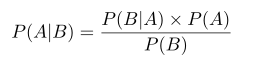In our case, the probability that we wish to calculate can be calculated as: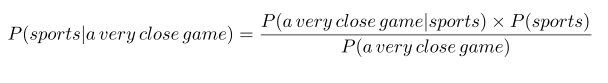Because we are only trying to find out which category (Sports or Not Sports) has a higher probability, it makes sense to discard the divisor P(a very close game), and compare only: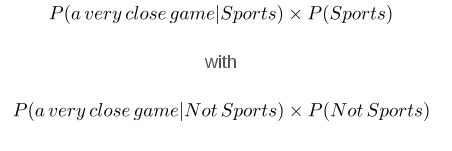But we have a problem: In order to obtain P(a very close game | Sports) , we have to count the occurrence of “a very close game” in the Sports category. But it does not even appear in the training set at all, thus the probability is zero, and consequently making P(a very close game | Sports) zero as well. What shall we do to tackle this problem?

Step 2: Being naive

In the non-naive Bayes way, we look at sentences in entirety, thus once the sentence does not show up in the training set, we will get a zero probability, making it difficult for further calculations. Whereas for Naive Bayes, there is an assumption that every word is independent of one another. Now, we look at individual words in a sentence, instead of the entire sentence.

Here, we can rewrite the probability we wish to calculate accordingly: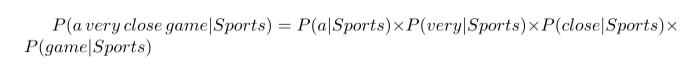Similarly,
P(a very close game | Not Sports) = P(a | Not Sports) x P(very | Not Sports) x P(close | Not Sports) x P(game | Not Sports)

Step 3: Calculating the probabilities

In the final step, we are good to go : simply calculating the probabilities and compare which has a higher probability: P(a very close game | Sports) or P(a very close game | Not Sports).

Here , the word “close” does not exist in the category Sports, thus P(close | Sports) = 0, leading to P(a very close game | Sports)=0. It is problematic when a frequency-based probability is zero, because it will wipe out all the information in the other probabilities, and we need to find a solution for this.

A solution would be Laplace smoothing , which is a technique for smoothing categorical data. A small-sample correction, or pseudo-count, will be incorporated in every probability estimate. Consequently, no probability will be zero. this is a way of regularizing Naive Bayes, and when the pseudo-count is zero, it is called Laplace smoothing. While in the general case it is often called Lidstone smoothing.

`In statistics, additive smoothing, also called Laplace smoothing (not to be confused with Laplacian smoothing), or Lidstone smoothing, is a technique used to smooth categorical data.`

` Given an observation x = (x1, …, xd) from a multinomial distribution with N trials and parameter vector θ = (θ1, …, θd), a "smoothed" version of the data gives the estimator:`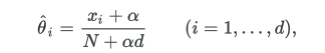`where the pseudocount α > 0 is the smoothing parameter (α = 0 corresponds to no smoothing). Additive smoothing is a type of shrinkage estimator, as the resulting estimate will be between the empirical estimate xi / N, and the uniform probability 1/d. Using Laplace's rule of succession, some authors have argued that α should be 1 (in which case the term add-one smoothing is also used), though in practice a smaller value is typically chosen.`

So how do we apply Laplace smoothing in our case?

We might consider setting the smoothing parameter α =1 and d=14 (see the equation above), we add 1 to every probability, therefore the probability, such as P(close | sports), will never be zero. And 14 is the number of possible words in two categories, making the division always greater than 1.

We can now calculate the probability again, this time it gives us a non-zero probability:

P(close | Sports ) =(0+1)/(11+14)= 1/25

Accordingly, we can obtain the other probabilities necessary for comparing P(a very close game | Sports) with P(a very close game | Not Sports):As seen from the results shown below, P(a very close game | Sports) gives a higher probability, suggesting that the sentence belongs to the Sports category.(Note that there is a typo in the second equation by the author, it should be P(a | Not Sports) … )

Finally, here are some advanced techniques for improving the basic Naive Bayes classifier: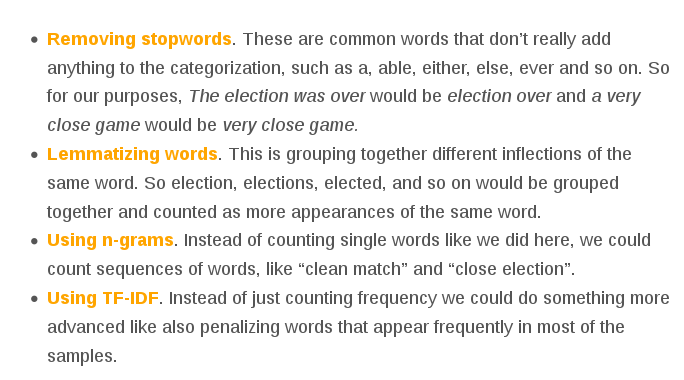When applying multinomial Naive Bayes to text classification problems, two questions that should be considered before getting started:

(1) Which features of text are you going to extract?

Feature engineering is a critical step when applying Naive Bayes classifiers. In Bruno’s blog post described above, he chose word frequency as the text features. However, to make your classifier more advanced, tf-idf makes an ideal option . Tf-idf not only counts the occurrence of a word in the given text(or document), but also reflect how important the word is to the document.

(2) Have you done smoothing on the probabilities?

The frequency-based probability might introduce zeros when multiplying the probabilities, leading to a failure in preserving the information contributed by the non-zero probabilities. Therefore, a smoothing approach, for example, the Laplace smoothing, must be adopted to counter this problem.

After deciding on these problems, you are more likely to obtain reasonable results when applying Naive Bayes classifiers. And you will find out that Naive Bayes classifiers are a good example of being both simple (naive) and powerful for NLP tasks such as text classification.

Author: Olli Huang | Technical Reviewer: Haojin Yang